# Quantum information and the monogamy of entanglement Aram

• Slides: 25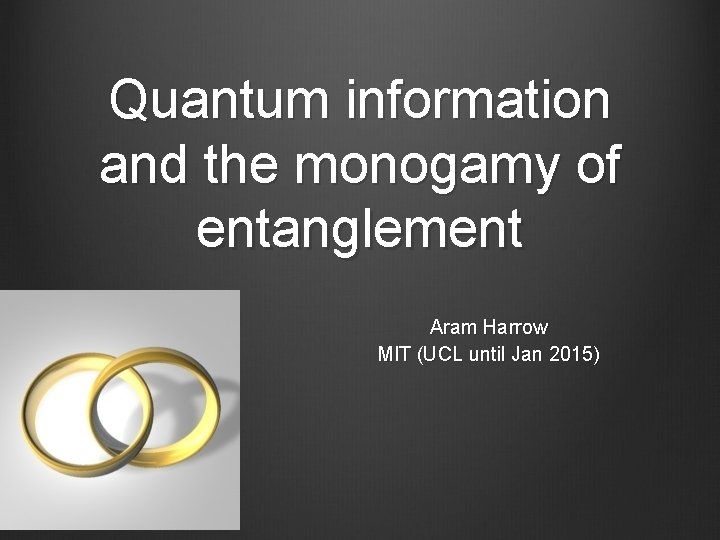Quantum information and the monogamy of entanglement Aram Harrow MIT (UCL until Jan 2015)Quantum mechanics Blackbody radiation paradox: How much power does a hot object emit at wavelength ¸? Classical theory (1900): const / ¸ 4 Quantum theory (1900 – 1924): Bose-Einstein condensate (1995) QM has also explained: • the stability of atoms • the photoelectric effect • everything else we’ve looked atDifficulties of quantum mechanics Heisenberg’s uncertainty principle Topological effects Entanglement Exponential complexity: Simulating N objects requires effort » exp(N)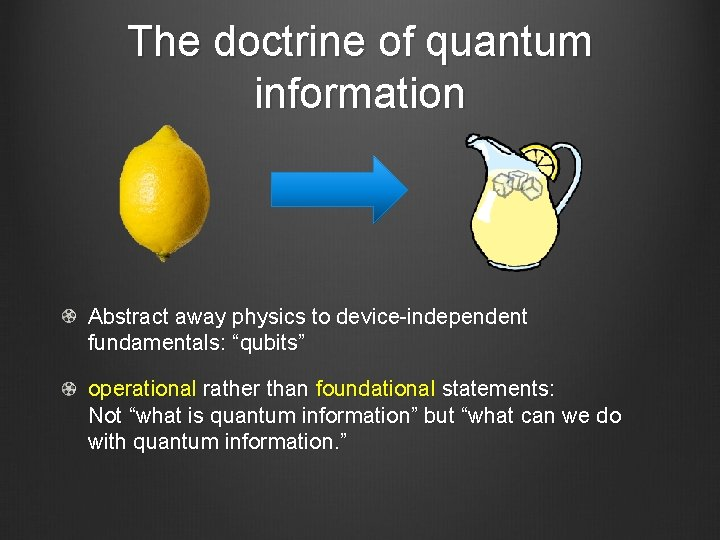The doctrine of quantum information Abstract away physics to device-independent fundamentals: “qubits” operational rather than foundational statements: Not “what is quantum information” but “what can we do with quantum information. ”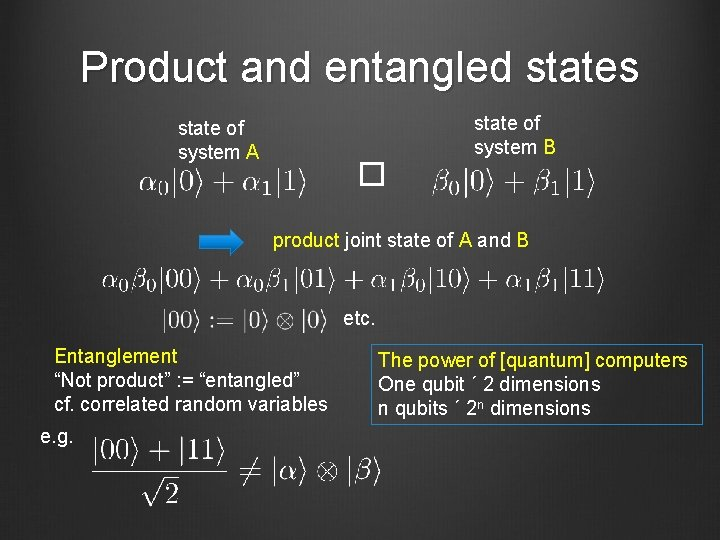Product and entangled states state of system A � state of system B product joint state of A and B etc. Entanglement “Not product” : = “entangled” cf. correlated random variables e. g. The power of [quantum] computers One qubit ´ 2 dimensions n qubits ´ 2 n dimensions[quantum] entanglement vs. [classical] correlation Make comparable using density matrices Entangled state Correlated state By contrast, a random mixture of |00 i and |11 i is How to distinguish? off-diagonal elements not enough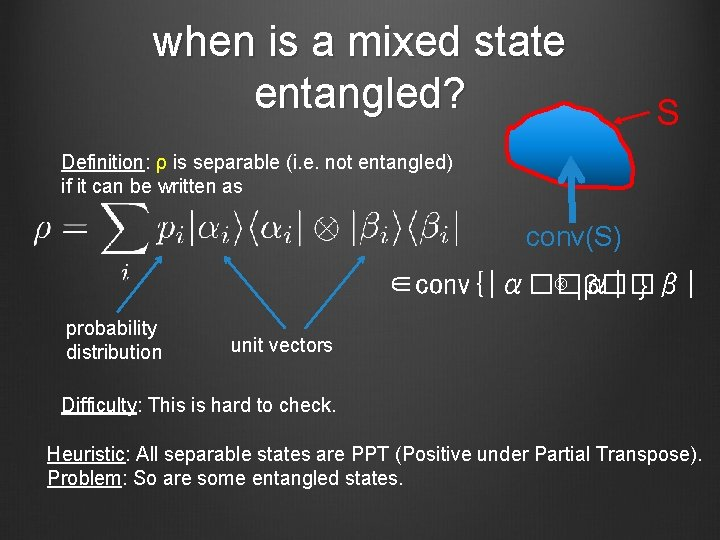when is a mixed state entangled? S Definition: ρ is separable (i. e. not entangled) if it can be written as conv(S) ∈conv{|α��α| ⊗ |β��β| } probability distribution unit vectors Difficulty: This is hard to check. Heuristic: All separable states are PPT (Positive under Partial Transpose). Problem: So are some entangled states.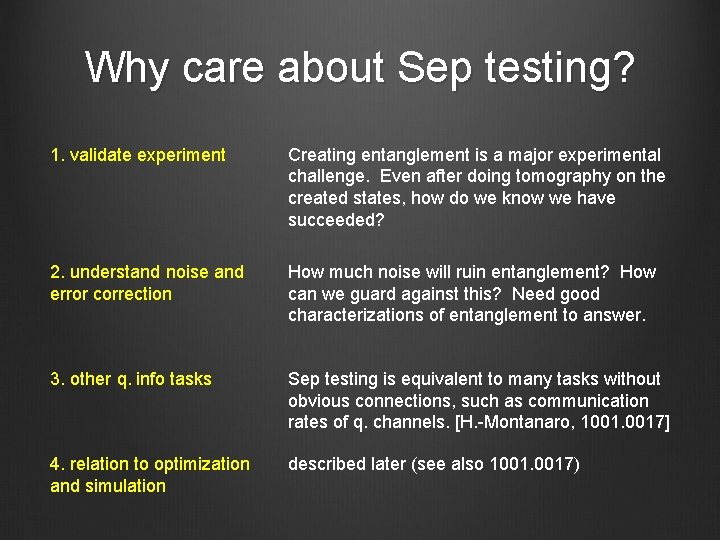Why care about Sep testing? 1. validate experiment Creating entanglement is a major experimental challenge. Even after doing tomography on the created states, how do we know we have succeeded? 2. understand noise and error correction How much noise will ruin entanglement? How can we guard against this? Need good characterizations of entanglement to answer. 3. other q. info tasks Sep testing is equivalent to many tasks without obvious connections, such as communication rates of q. channels. [H. -Montanaro, 1001. 0017] 4. relation to optimization and simulation described later (see also 1001. 0017)limits on entanglement testing Detecting pure-state entanglement is easy, so detecting mixed-state entanglement is hard [H-Montanaro, 1001. 0017] 1. Given , how close is |ψ� to a state of the form |α 1� � |α 2� � …�|α N�? 2. With one copy of |ψ� this is impossible to estimate. We give a simple test that works for two copies. 3. Combine this with a) [Aaronson-Beigi-Drucker-Fefferman-Shor 0804. 0802] b) a widely believed assumption (the “exponential time hypothesis”) c) other connecting tissue (see our paper) to prove that testing whether a d-dimensional state is approximately separable requires time ≥ dlog(d). 4. This rules out any simple heuristic (e. g. checking eigenvalues).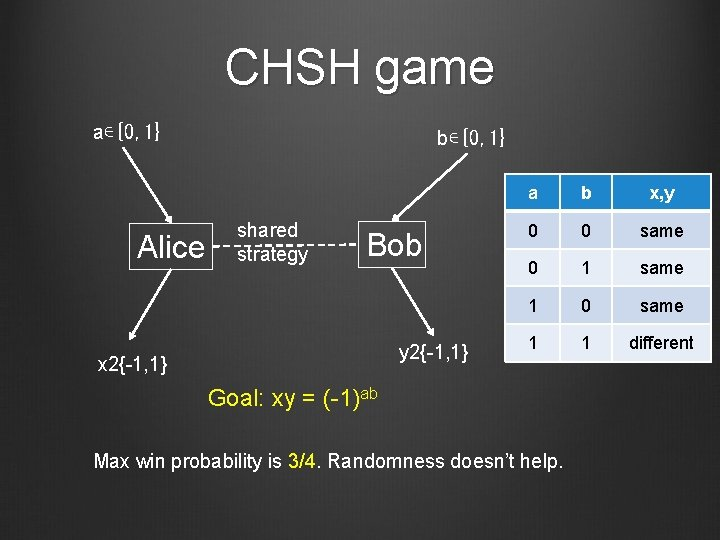CHSH game a∊{0, 1} Alice b∊{0, 1} shared strategy Bob y 2{-1, 1} x 2{-1, 1} a b x, y 0 0 same 0 1 same 1 0 same 1 1 different Goal: xy = (-1)ab Max win probability is 3/4. Randomness doesn’t help.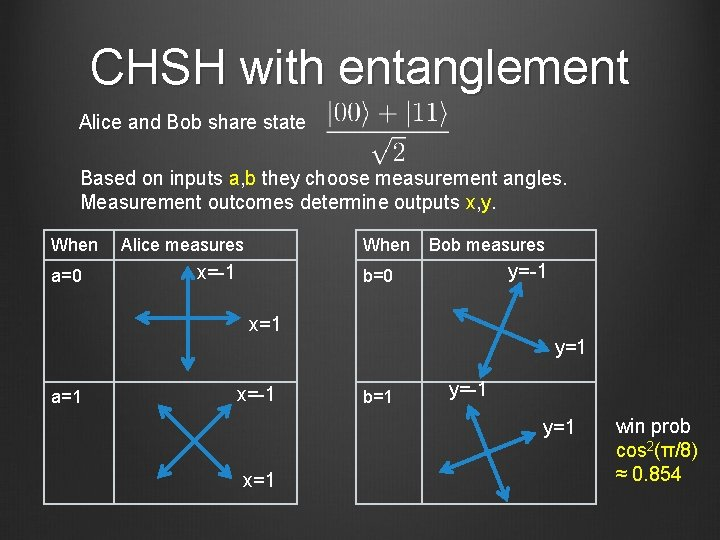CHSH with entanglement Alice and Bob share state Based on inputs a, b they choose measurement angles. Measurement outcomes determine outputs x, y. When a=0 Alice measures When x=-1 Bob measures y=-1 b=0 x=1 a=1 x=-1 y=1 b=1 y=-1 y=1 x=1 win prob cos 2(π/8) ≈ 0. 854CHSH with entanglement a=0 x=-1 b=0 y=-1 a=1 x=-1 goal: xy=(-1)ab b=1 y=1 a=0 x=1 b=0 y=1 a=1 x=1 b=1 y=-1 Why it works Winning pairs are at angle π/8 Losing pairs are at angle 3π/8 2(π/8) ∴ Pr[win]=cos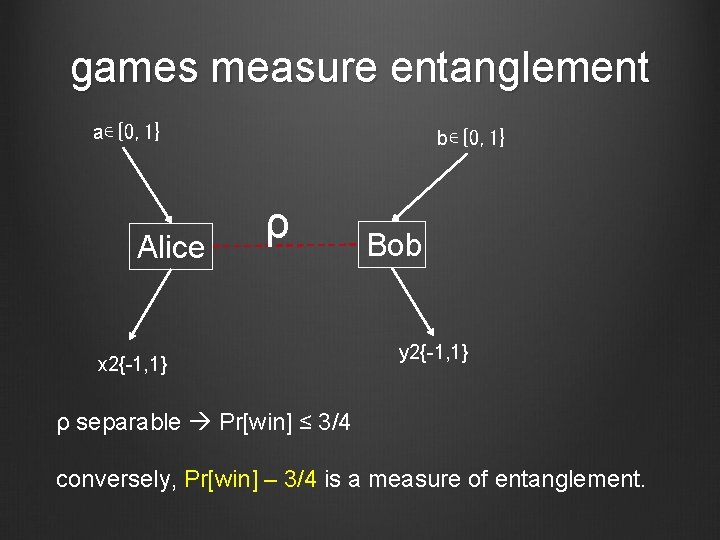games measure entanglement a∊{0, 1} Alice b∊{0, 1} ρ x 2{-1, 1} Bob y 2{-1, 1} ρ separable Pr[win] ≤ 3/4 conversely, Pr[win] – 3/4 is a measure of entanglement.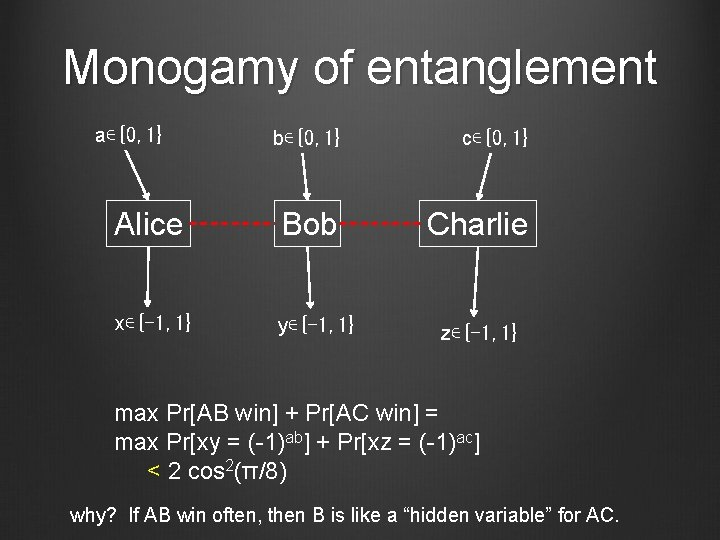Monogamy of entanglement a∊{0, 1} b∊{0, 1} c∊{0, 1} Alice Bob Charlie x∊{-1, 1} y∊{-1, 1} z∊{-1, 1} max Pr[AB win] + Pr[AC win] = max Pr[xy = (-1)ab] + Pr[xz = (-1)ac] < 2 cos 2(π/8) why? If AB win often, then B is like a “hidden variable” for AC.shareability implies separability a b 1 b 2 A B 1 B 2 x y 1 y 2 bk … Bk yk CHSH any game Intuition: Measuring B 2, …, Bk leaves A, B 1 nearly separable Proof uses information theory: [Brandão-H. , 1210. 6367, 1310. 0017] 1. conditional mutual information shows game values monogamous 2. other tools show “advantage in non-local games” ≈ “entanglement”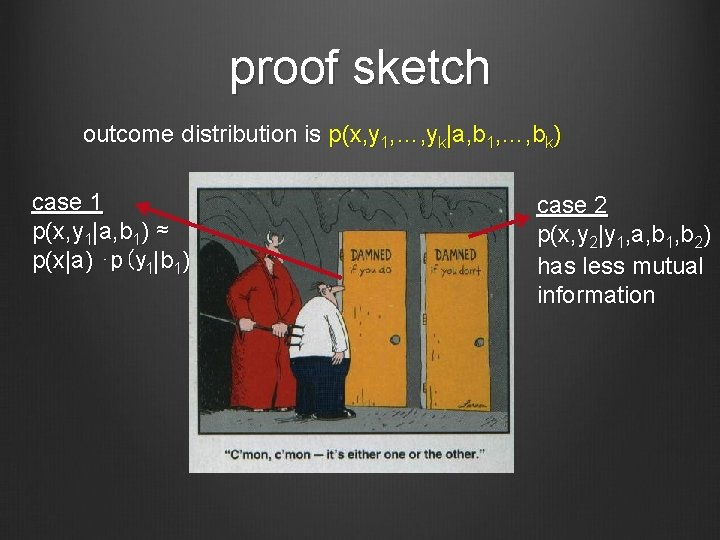proof sketch outcome distribution is p(x, y 1, …, yk|a, b 1, …, bk) case 1 p(x, y 1|a, b 1) ≈ p(x|a) ⋅p(y 1|b 1) case 2 p(x, y 2|y 1, a, b 1, b 2) has less mutual information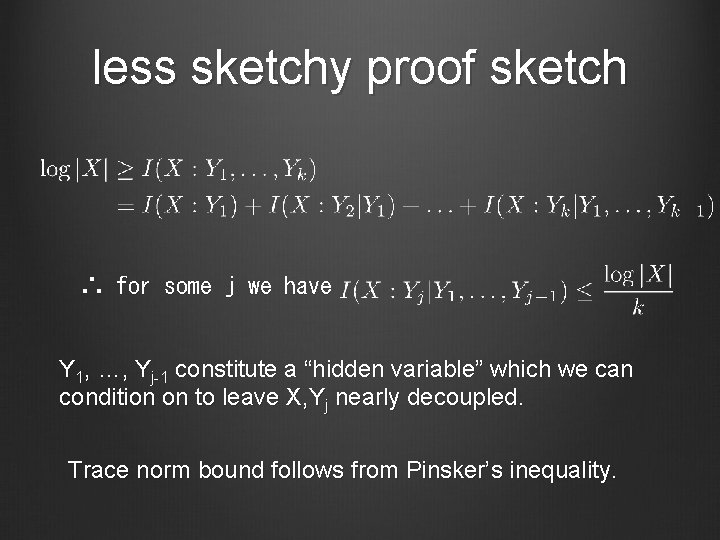less sketchy proof sketch ∴ for some j we have Y 1, …, Yj-1 constitute a “hidden variable” which we can condition on to leave X, Yj nearly decoupled. Trace norm bound follows from Pinsker’s inequality.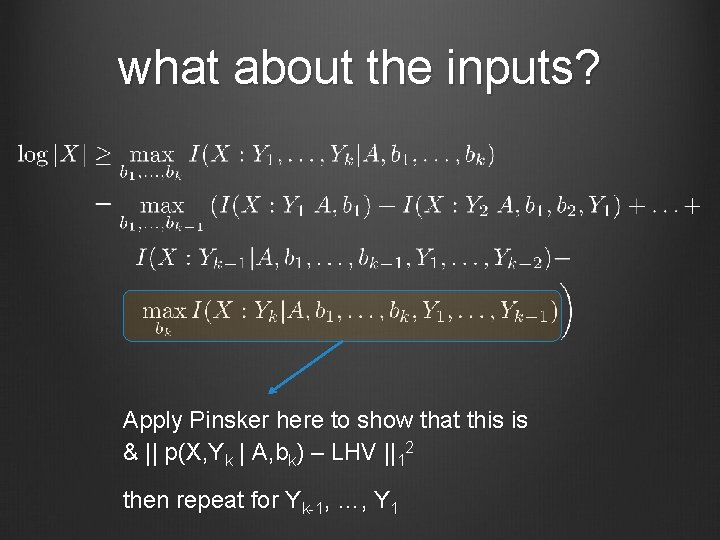what about the inputs? Apply Pinsker here to show that this is & || p(X, Yk | A, bk) – LHV ||12 then repeat for Yk-1, …, Y 1A hierachy of tests for entanglement Definition: ρAB is k-extendable if there exists an extension with for each i. all quantum states (= 1 -extendable) 2 -extendable 100 -extendable separable = ∞-extendable Algorithms: Can search/optimize over k-extendable states in time d. O(k). Question: How close are k-extendable states to separable states?application #1: mean-field approximation ∞-D used in limit of high coordination number, e. g. 1 -D 2 -D Bethe lattice 3 -Dmean-field product states mean-field ansatz for homogenous systems: for inhomogenous systems: ⊗N |α� |α 1��|α 2��…�|α N� Result: Controlled approximation to ground-state energy with no homogeneity assumptions based only on coordination number. [Brandão-H. 1310. 0017] Application: “No low-energy trivial states” conjecture [Freedman-Hastings] states that there exist Hamiltonians where all low-energy states have topological order. ∴ This can only be possible with low coordination number.application #2: optimization connections to: • polynomial opt. • unique games conjecture Given a Hermitian matrix M: • maxα hα| M |αi is easy • maxα, β hα�β | M |α�β i is hard Approximate with A Computational effort: d. O(k) B 1 B 2 B 3 B 4 Key question: approximation error as a function of k and d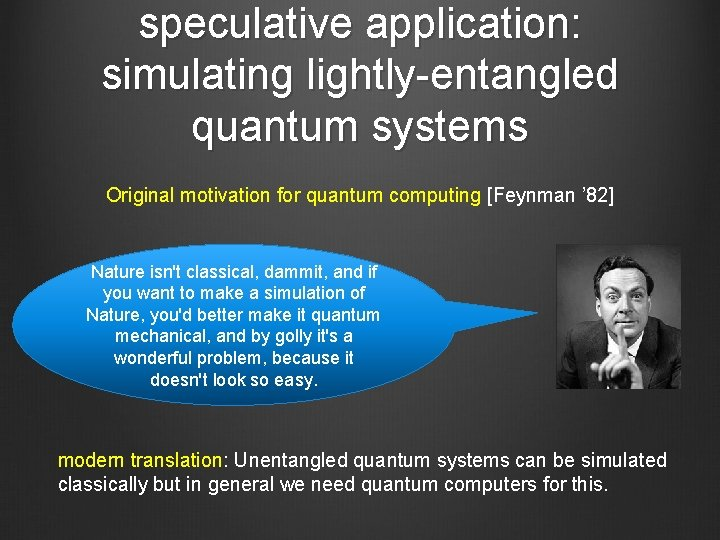speculative application: simulating lightly-entangled quantum systems Original motivation for quantum computing [Feynman ’ 82] Nature isn't classical, dammit, and if you want to make a simulation of Nature, you'd better make it quantum mechanical, and by golly it's a wonderful problem, because it doesn't look so easy. modern translation: Unentangled quantum systems can be simulated classically but in general we need quantum computers for this.low-entanglement simulation degree of entanglement classically simulatable supports universal classical computing Open question: Are there good classical simulations of lightly-entangled quantum systems? ? supports universal quantum computing Idea: model k-body ≈0. 1 ns single-qubit Rabi oscillations reduced density matrices ≈2. 5 ns decoherence time where k scales with ≈10µs computation time entanglement. cf. results for ground states in 1310. 0017.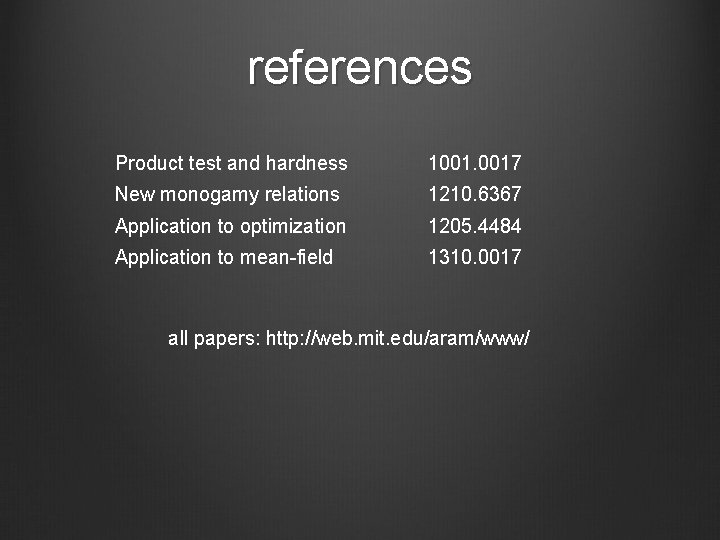references Product test and hardness 1001. 0017 New monogamy relations 1210. 6367 Application to optimization 1205. 4484 Application to mean-field 1310. 0017 all papers: http: //web. mit. edu/aram/www/